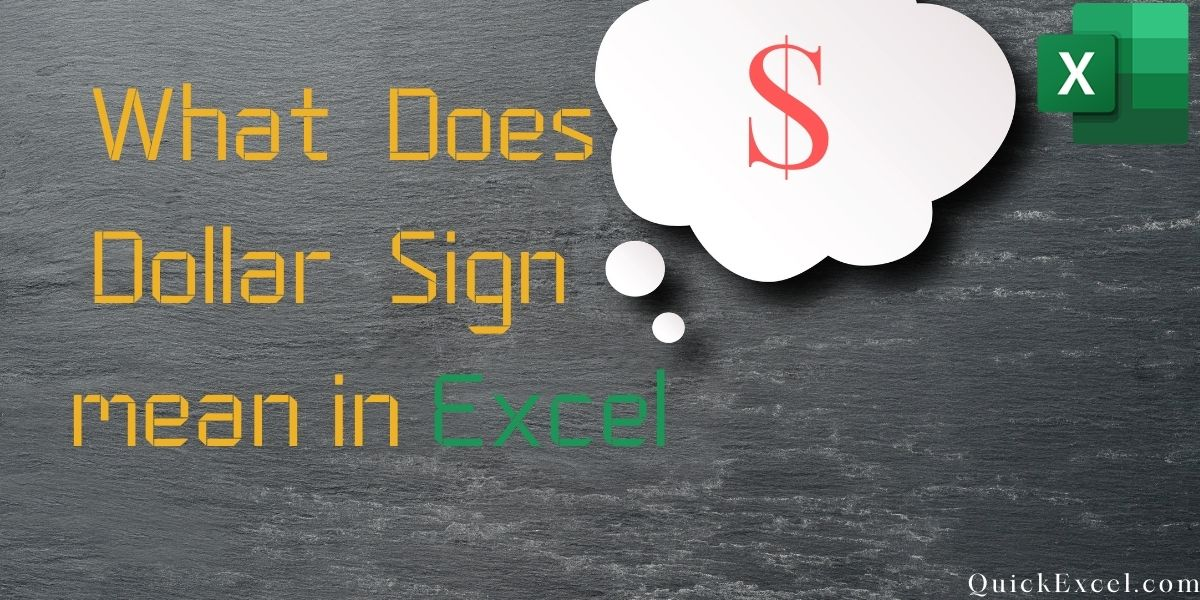# Dollar Sign in Excel: What does Dollar Sign (\$) mean in Excel?In this article, we’ll learn what the dollar sign in Excel stands for. The ability to refer to cells or ranges and use them in certain formulas is one of the features that makes Excel a valuable tool. These cell references may automatically alter when you copy these formulas. In the example below, we copied cell C2, which contains a formula, and pasted it into cell C3.

You can see how the formula changes the given references when you copy-paste the cells. While cell C2 contains the formula for A2 and B2, cell C3 contains the formula for A3 and B3.

This is known as a relative reference, it adjusts the references based on the cells to which it is applied.

If you put a \$ symbol before a cell reference, for example, \$C\$2, Excel knows it refers to cell C3 even if you copy or paste the calculation. So, there are three various types of references on Excel now that you can utilize the dollar (\$) sign in three different forms.

## Inserting A (\$) Dollar sign in Excel To Cell Reference In Shortcut

In Microsoft Excel, you can add the dollar (\$) symbol to a cell reference in two ways.

Type 1 – You can get there manually, in other words, go in the edit mode in the cell by clicking twice on it or by pressing F2, place the pointer where you’d like the \$ sign, and then type it manually or you could just so it automatically i.e., go in the edit menu in a cell by double-clicking on it or by using the F2 key, place the cursor where you would like to insert the \$ sign, and afterward manually type it.

Type 2 – you can also use the F4 key as a shortcut. Simply position the cursor in the cell reference wherever you want to put the dollar \$ sign and click it once to apply this shortcut. It will alter the reference just by adding or deleting the dollar \$ symbol.

Consider the case where you have the references C2 in the cell. The F4 shortcut will function as follows:

• If you press F4 once, C2 will become \$C\$2.
• C2 will become C\$2 if you press F4 twice.
• C2 will become \$C2 if you press F4 thrice.
• C2 will revert to C2 if you press F4 four times.

## Types Of References In Excel

In Microsoft excel, there are in total three types of references:

### 1. Relative Reference

When you use a relative reference in excel, you wouldn’t use the dollar sign at all. Whenever you copy a cell with a relative reference, it will modify and adapt to the cell where it is copied. The references are adjusted as fast as the cell with the formula is copied and pasted.

### 2. Absolute Reference

The dollar \$ sign comes before the row number and column alphabet in the absolute reference, for example – \$C\$3. This will not modify the references when it is used in the formulas. When a cell is copied and pasted, and you have a value that must remain constant, this could be constant.

### 3. Mixed Reference

You only use the dollar \$ sign once in mixed reference, for example, \$C3 or C\$3. These references are more complicated than the relative and absolute references.

Here’s a quick rundown of what the \$ sign in excel formula means –

• \$A\$1 – Always links to row 1 and column A.
• \$A1 – column A is set and fixed and would not change. However, the row can be changed when the formulas are copied.
• A\$1 – the first row is fixed and would not transform, but the column can change when the formula is copied.
• The range A1:A100 is always denoted by \$A\$1:\$A\$100.

## Conclusion

That’s It! We hope you learned and enjoyed this lesson on using the dollar sign in Excel, stay tuned and we’ll be back soon with another awesome Excel tutorial at QuickExcel!